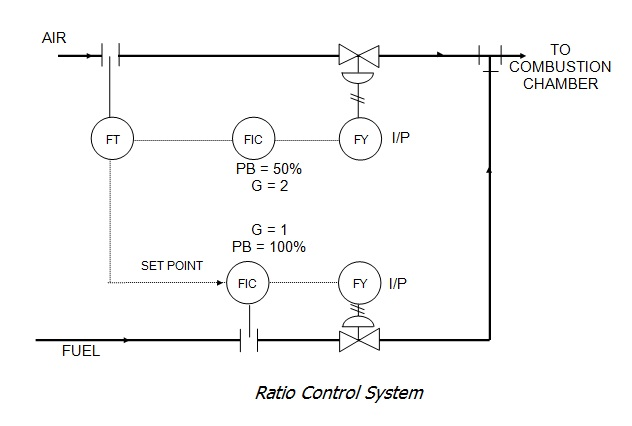# WHAT IS RATIO CONTROL ?## RATIO CONTROL

A ratio controller is a special type of feed forward controller where disturbances are measured and their ratio is held at a desired set point by controlling one of the streams.Ratio control is used when two fluids must be mixed together in a specific ratio.  A practical way to do this is to use a standard control system to control the flow on one line.  The same transmitter signal is used as a set point for a second controller which controls the flow in a second line.  The ratio of one flow rate to the other can be changed by adjusting the gain (or proportional band) of the secondary controller.  Figure shows a typical ratio control system.  The air to fuel ratio of the fluid going to the combustion chamber is set at 2:1.

### Applying Ratio Control

You can get a much better grasp of ratio control by considering some specific examples. Assume we want a “blender,” that is, to blend two streams, A and B, in a proportion or ratio R:

### R=B/A

An example for ratio control is given in figure below, the wild flow A is measured, and then it is multiplied by R to get the required value of B. Thus, as the flow A varies, the set point to the flowcontroller of stream B will vary to maintain R. If a new value of R is desired, it must be set into the ratio station. Differential-pressure sensors are shown measuring flow; their output indicates the square of flow and, therefore, square root extractors are shown to obtain flow. By using flow, and not its square, the loops will behave more linearly and thus will be more stable and easier to tune.